Sunday 20th September 2020

CBSE Guess > Papers > Question Papers > Class XII > 2003 > Mathematics > Delhi Set -II

MATHEMATICS—2003 (Set II—Delhi)

Note: Except for the following questions, all the remaning questions have been asked in Set I. D. B., 2003.

SECTION - A

Q. 3. Differentiate logQ. 7. Evaluate:Q. 10. Prove that: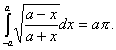Q. 14. In a correlation analysis, the following values are obtained about the variables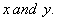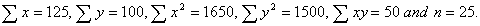Find the equations of line of regression of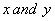and the value of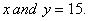Q. 17. Using matrix method, solve the following equations: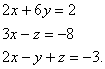SECTION - B

Q. 19. Three forces of magnitude P, Q and R acting at a point are in equilibrium. The angle between P and Q is 1200 and between P and R is 90 0 . Show that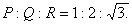Q. 20. A train moving with a velocity of 90 km/hr is brought to rest in 3 minutes by applying brakes. Calculate the retardation produced and the distance traveled by the before it comes to rest.

Q. 24. Solve the following differentia equation:SECTION - C

Q. 19. The first three moments of a distribution about the value 2 of the variable are 1, 16 and -40 respectively. Calculate the mean, variance and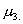Also calculate the first three moments about the origin.

Q. 21. An exchange broker converted Rs. 1,00,000 into pound sterling when the Mumbai - London rate was 1.66 pence and sold out the proceeds when the rate fell to 1.62 pence. Find the percentage profit.

Q. 24. A, B and C entered into a business and contributed Rs. 36,000, Rs. 60,000 and Rs. 30,000 respectively. They had the understanding that after allowingof the profit to C as a Manager, the remainder will be shared amongst them in proportion to their contribution towards the capital. At the end of the year, C received Rs. 8,000. What was the total profit and how much did the other partners receive?

 Mathematics 2003 Question Papers Class XII Delhi Outside Delhi Compartment Delhi Compartment Outside DelhiSet ISet ISet ISet ISet IISet IISet IISet IISet IIISet III

CBSE 2003 Question Papers Class XII